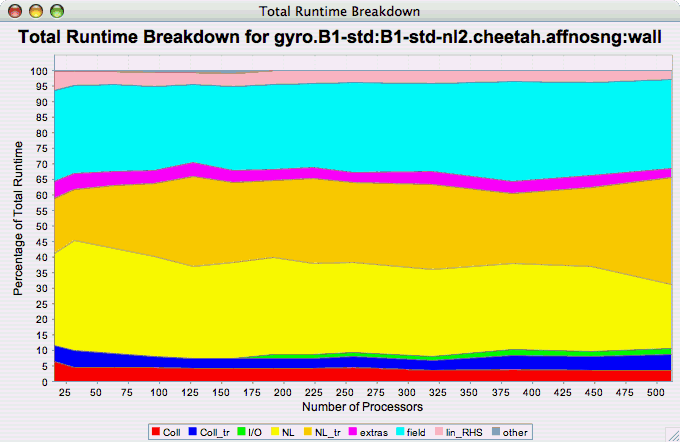## 22.2. Standard Chart Types

### 22.2.1. Timesteps Per Second

The Timesteps Per Second chart shows how an application scales as it relates to time-to-solution. If the timesteps are not already set, you will be prompted to enter the total number of timesteps in the trial (see Section 22.1.4, “Total Number of Timesteps” ). If there is more than one metric to choose from, you may be prompted to select the metric of interest (see Section 22.1.2, “Metric of Interest”). To request this chart, select one or more experiments or one view, and select this chart item under the "Charts" main menu item.

Figure 22.5. Timesteps per Second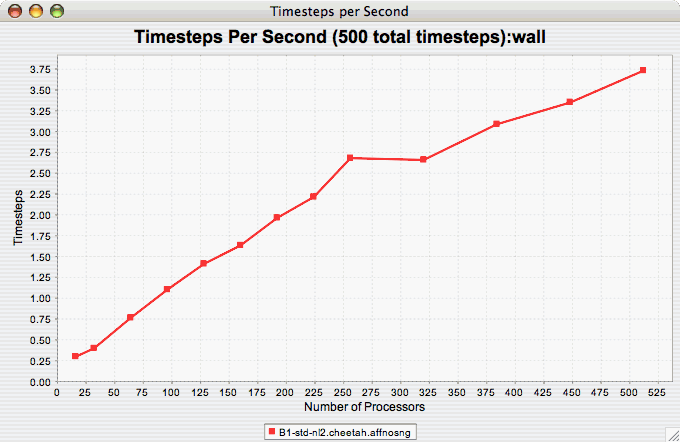### 22.2.2. Relative Efficiency

The Relative Efficiency chart shows how an application scales with respect to relative efficiency. That is, as the number of processors increases by a factor, the time to solution is expected to decrease by the same factor (with ideal scaling). The fraction between the expected scaling and the actual scaling is the relative efficiency. If there is more than one metric to choose from, you may be prompted to select the metric of interest (see Section 22.1.2, “Metric of Interest”). To request this chart, select one experiment or view, and select this chart item under the "Charts" main menu item.

Figure 22.6. Relative Efficiency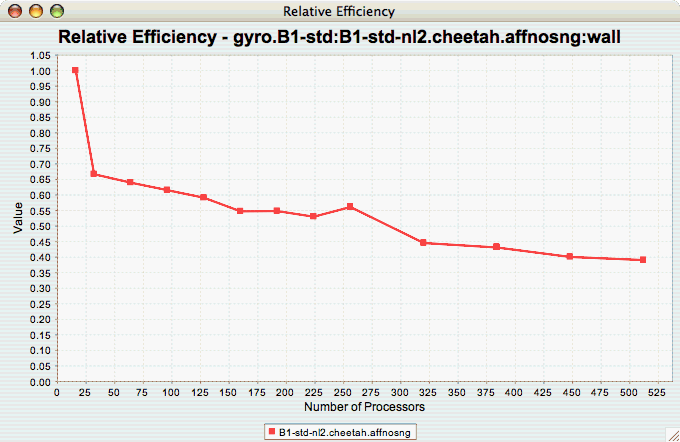### 22.2.3. Relative Efficiency by Event

The Relative Efficiency By Event chart shows how each event in an application scales with respect to relative efficiency. That is, as the number of processors increases by a factor, the time to solution is expected to decrease by the same factor (with ideal scaling). The fraction between the expected scaling and the actual scaling is the relative efficiency. If there is more than one metric to choose from, you may be prompted to select the metric of interest (see Section 22.1.2, “Metric of Interest”). To request this chart, select one or more experiments or one view, and select this chart item under the "Charts" main menu item.

Figure 22.7. Relative Efficiency by Event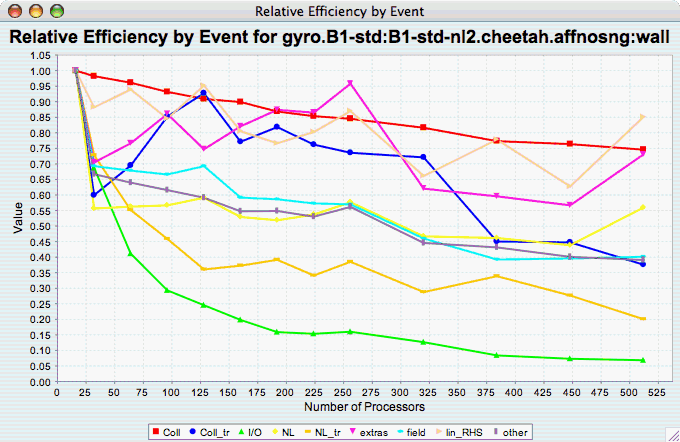### 22.2.4. Relative Efficiency for One Event

The Relative Efficiency for One Event chart shows how one event from an application scales with respect to relative efficiency. That is, as the number of processors increases by a factor, the time to solution is expected to decrease by the same factor (with ideal scaling). The fraction between the expected scaling and the actual scaling is the relative efficiency. If there is more than one event to choose from, and you have not yet selected an event of interest, you may be prompted to select the event of interest (see Section 22.1.3, “Event of Interest”). If there is more than one metric to choose from, you may be prompted to select the metric of interest (see Section 22.1.2, “Metric of Interest”). To request this chart, select one or more experiments or one view, and select this chart item under the "Charts" main menu item.

Figure 22.8. Relative Efficiency one Event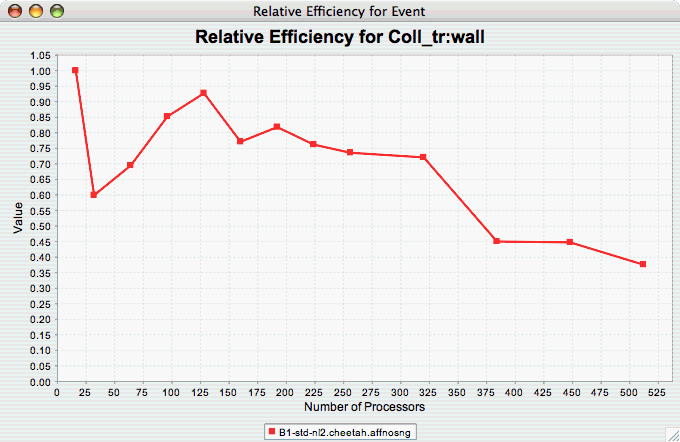### 22.2.5. Relative Speedup

The Relative Speedup chart shows how an application scales with respect to relative speedup. That is, as the number of processors increases by a factor, the speedup is expected to increase by the same factor (with ideal scaling). The ideal speedup is charted, along with the actual speedup for the application. If there is more than one metric to choose from, you may be prompted to select the metric of interest (see Section 22.1.2, “Metric of Interest”). To request this chart, select one or more experiments or one view, and select this chart item under the "Charts" main menu item.

Figure 22.9. Relative Speedup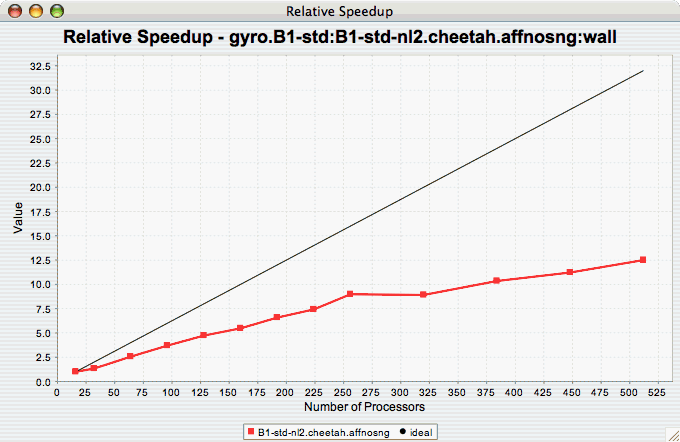### 22.2.6. Relative Speedup by Event

The Relative Speedup By Event chart shows how the events in an application scale with respect to relative speedup. That is, as the number of processors increases by a factor, the speedup is expected to increase by the same factor (with ideal scaling). The ideal speedup is charted, along with the actual speedup for the application. If there is more than one metric to choose from, you may be prompted to select the metric of interest (see Section 22.1.2, “Metric of Interest”). To request this chart, select one experiment or view, and select this chart item under the "Charts" main menu item.

Figure 22.10. Relative Speedup by Event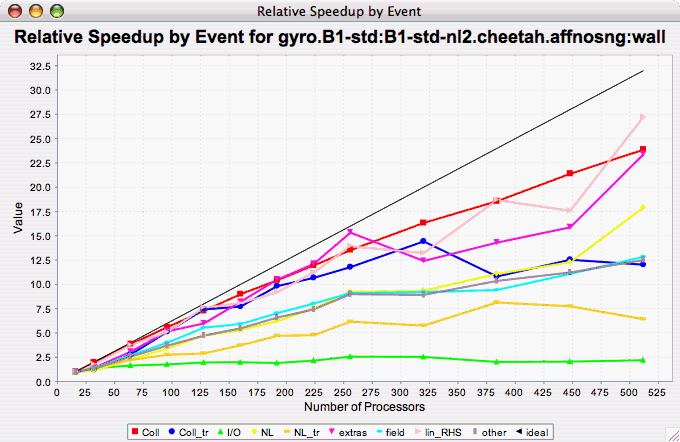### 22.2.7. Relative Speedup for One Event

The Relative Speedup for One Event chart shows how one event in an application scales with respect to relative speedup. That is, as the number of processors increases by a factor, the speedup is expected to increase by the same factor (with ideal scaling). The ideal speedup is charted, along with the actual speedup for the application. If there is more than one event to choose from, and you have not yet selected an event of interest, you may be prompted to select the event of interest (see Section 22.1.3, “Event of Interest”). If there is more than one metric to choose from, you may be prompted to select the metric of interest (see Section 22.1.2, “Metric of Interest”). To request this chart, select one or more experiments or one view, and select this chart item under the "Charts" main menu item.

Figure 22.11. Relative Speedup one Event### 22.2.8. Group % of Total Runtime

The Group % of Total Runtime chart shows how the fraction of the total runtime for one group of events changes as the number of processors increases. If there is more than one group to choose from, and you have not yet selected a group of interest, you may be prompted to select the group of interest (see Section 22.1.1, “Group of Interest”). If there is more than one metric to choose from, you may be prompted to select the metric of interest (see Section 22.1.2, “Metric of Interest”). To request this chart, select one or more experiments or one view, and select this chart item under the "Charts" main menu item.

Figure 22.12. Group % of Total Runtime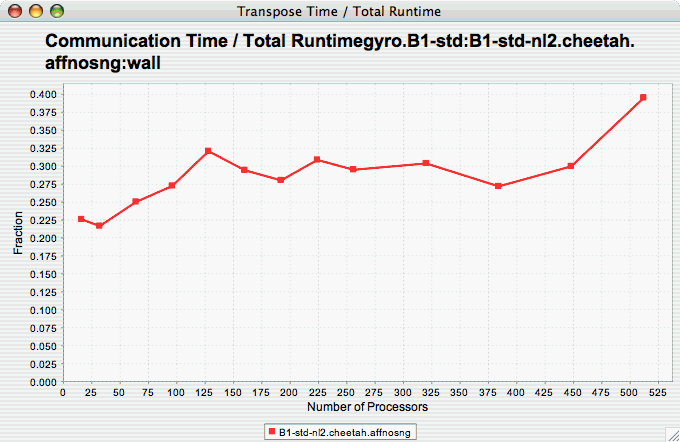### 22.2.9. Runtime Breakdown

The Runtime Breakdown chart shows the fraction of the total runtime for all events in the application, and how the fraction changes as the number of processors increases. If there is more than one metric to choose from, you may be prompted to select the metric of interest (see Section 22.1.2, “Metric of Interest”). To request this chart, select one experiment or view, and select this chart item under the "Charts" main menu item.

Figure 22.13. Runtime Breakdown